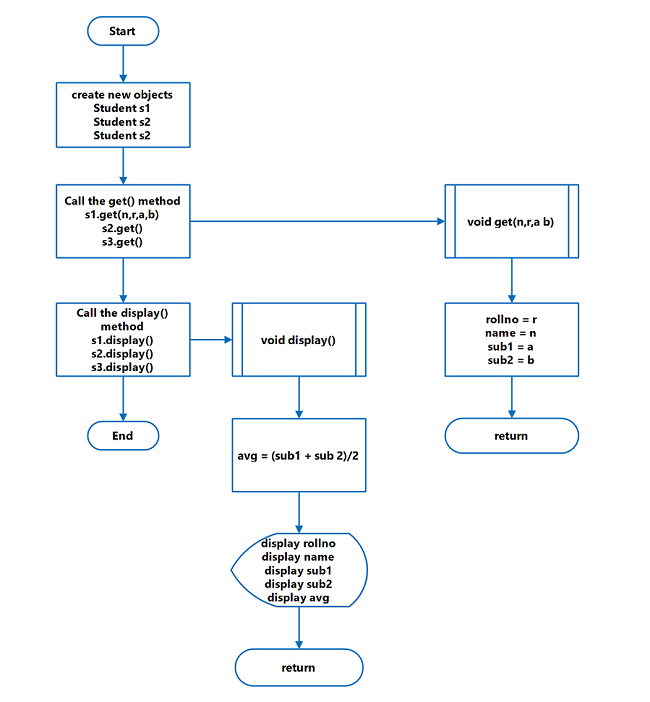## Java Program to Compute Average Mark of Students

The program answers how to compute average mark of student. It reads a student’s mark in each subject and then compute the average and displays the results.

First, you will store the student records and second, take the average of the marks obtained in different subjects. This is a simple program that demonstrates the use of arithmetic expressions in a java program. The java program to calculate student marks is intended for beginners of java programming.

A flowchart, program code and a verified output is given to help you learn and practice the program. The program is written using NetBeans 8.2 with Java SDK 8u111.

## Problem Definition

The program for computing average mark of students ask for student’s name, roll number and marks in two subjects – sub1 and sub2 using get() function. We have used only two subjects for the program, but you can always change the number of the subject suitable to you.

This will not break the program logic and still calculate average.

Next, the program computes the total marks and divide it with the number of subjects taken by the student.

This will give the average mark obtained by the student. To compute the average use following

``Average mark = Total marks all subjects/Total subjects``

Finally, the program displays the results using function – disp().

## Flowchart – Average Mark of StudentsFlowchart – Average Mark of Student

## Program Code – Average Mark of Student

``````/* * To change this license header, choose License Headers in Project Properties.
* To change this template file, choose Tools | Templates
* and open the template in the editor. */

package JavaExamples;

/** * * @author Admin */

class Student{

int rollno;
String name;
int sub1;
int sub2;
int Avg;
void get(String n, int r, int a, int b) {
rollno = r;
name = n;
sub1 = a;
sub2 = b;
}

void disp() {
Avg = (sub1 + sub2)/2;
System.out.println("Rollno=" + rollno);
System.out.println("Name=" + name);
System.out.println("Sub1=" + sub1);
System.out.println("Sub2=" + sub2);
System.out.println("Average=" + Avg);
}
}

public class StudentMarkAvg {
public static void main(String[] args){
Student s1 = new Student();
Student s2 = new Student();
Student s3 = new Student();
s1.get("Ram",16345,21,78);
s2.get("Judith", 26312, 77,91);

s3.get("Lee", 32132, 85, 65);
s1.disp();
s2.disp();
s3.disp();
}
}``````

## Output – Average Mark

The output of the program compute average mark of student in NetBeans 8.2 is given below. It displays the student details and the average mark in two subjects.

``````
Rollno=16345

Name=Ram

Sub1=21

Sub2=78

Average=49

------------------------------

Rollno=26312

Name=Judith

Sub1=77

Sub2=91

Average=84

------------------------------

Rollno=32132

Name=Wong

Sub1=85

Sub2=65

Average=75

------------------------------

``````